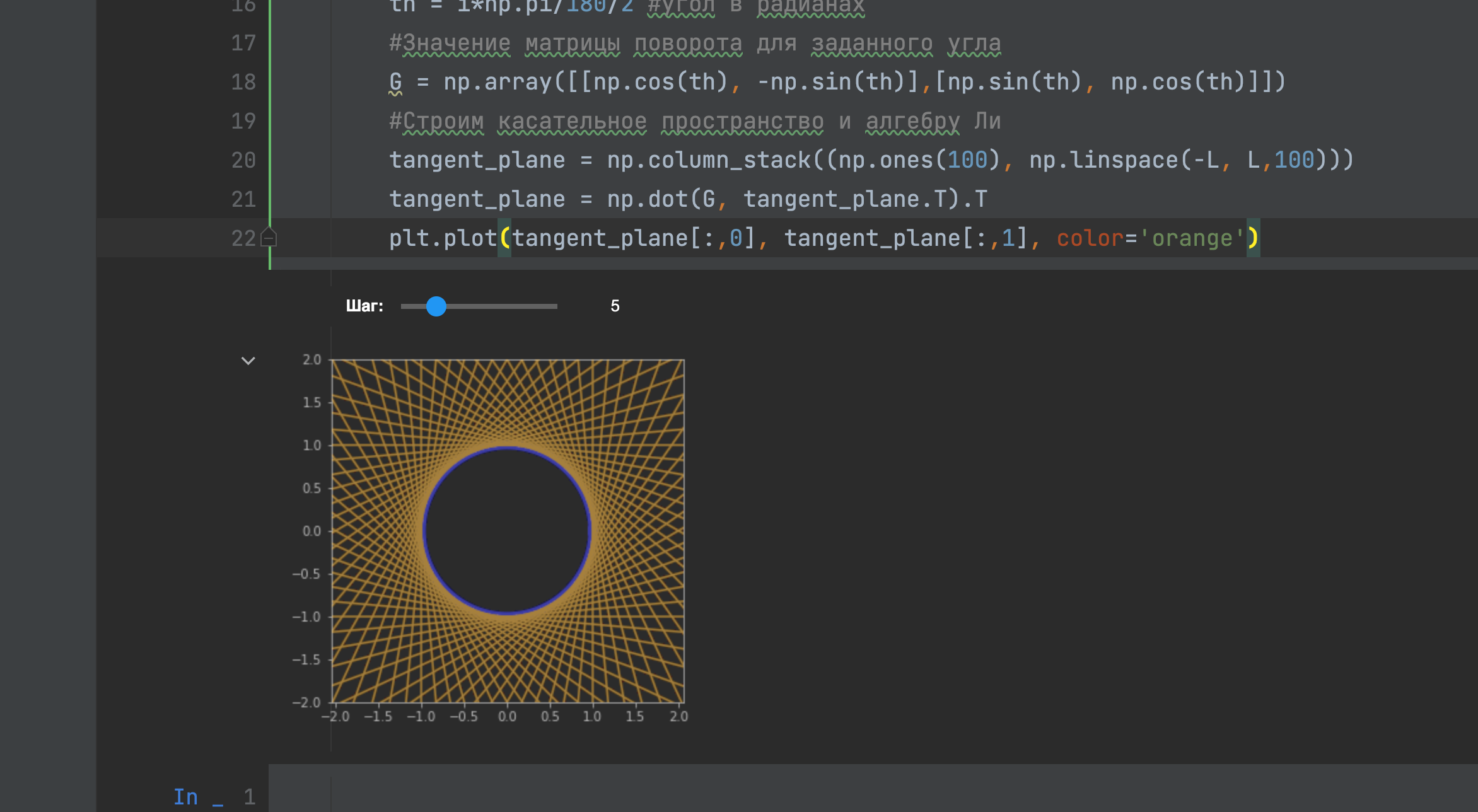Instead of a chart, PyCharm outcome is <Figure size 1000x1000 with 1 Axes>. The Jupyter Notebook (jupyter.org) displays the chart correctly. Plt.show() doesn't help. %matplotlibe inline is before importing the matplotlib library.Which PyCharm version are you using?

Could you please provide a code sample to reproduce the issue?I use the last version of PyCharm Professional (2021.3.1).

Here is some code example:

`%matplotlib inlineimport randomimport numpy as npimport matplotlib.pyplot as pltimport ipywidgets as widgets`
`_step = widgets.IntSlider(min=1,max=20,step=1,continuous_update=False,description='Шаг:',value=10,orientation='horizontal',readout=True)`
`#Интерактивное управление параметрами модели с помощью виджетов@widgets.interact(step = _step)def plot(step=1):    #размер окна    plt.figure(figsize=(5, 5))    plt.axis('equal')    plt.grid(False)    plt.axis((-2, 2, -2, 2))    #изображаем множество точек, принадлежащих S1    theta = np.linspace(0, 2*np.pi, 100)    plt.plot(np.cos(theta), np.sin(theta), color='blue', linewidth=7)    S = 2 #задаем масштаб по осям    L=5 #длина изображаемого участка касательной и алгебры Ли    for i in range(0, 360*2, step):        th = i*np.pi/180/2 #угол в радианах        #Значение матрицы поворота для заданного угла        G = np.array([[np.cos(th), -np.sin(th)],[np.sin(th), np.cos(th)]])        #Строим касательное пространство и алгебру Ли        tangent_plane = np.column_stack((np.ones(100), np.linspace(-L, L,100)))        tangent_plane = np.dot(G, tangent_plane.T).T        plt.plot(tangent_plane[:,0], tangent_plane[:,1], color='orange')`Thank you,

There is a known issue with ipywidgets - https://youtrack.jetbrains.com/issue/DS-2766

Though the widget doesn't work, the graph is displayed correctly in my case, using your code sample:If the issue is still relevant, could you please let me know your python version, and the output of `pip freeze` ?I found that matplotlib needed to have a ENV variable set or this code
matplotlib.use('TkAgg')
to show the plot window on windows10 in pycharm.